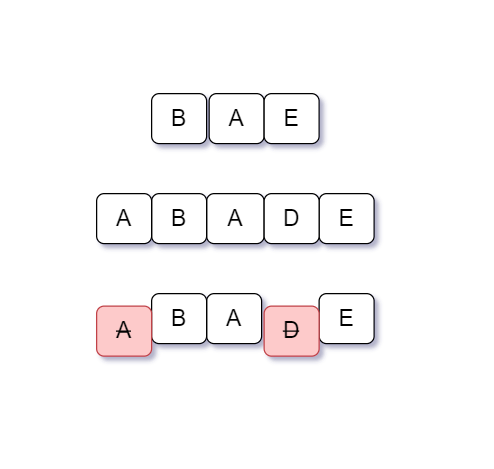New update is available. Click here to update.

# Is SubSequence

Last Updated: 18 Nov, 2020
Difficulty: Easy

## PROBLEM STATEMENT

#### A subsequence of a string is a new string that can be derived from the original string by deleting some characters (can be none) without changing the relative ordering of other characters.

##### Example:
``````‘ACE’ is a subsequence of ‘ABCDE’ because ‘ACE’ can be formed by deleting ‘B’ and ‘D’ without changing the relative order of characters. ‘ADB’ is not a subsequence of ‘ABCDE’ because we can get ‘ABD’ from ‘ABCDE’ but not ‘ADB’ and in ‘ADB’ relative order of ‘B’ and ‘D’ are different from original strings.
``````
##### Note:
``````1.Strings ‘STR1’ and ‘STR2’ consists only of English uppercases.

2.Length of string ‘STR2’ will always be greater than or equal to the length of string ‘STR1’.
``````

#### Example:

``````For example, the given ‘STR1’ is ‘BAE’ and ‘STR2’ is ‘ABADE’.
String ‘STR1’ is a subsequence of string ‘STR2’ because ‘BAE’ can be formed by deleting ‘A’ and ‘D’ from ‘ABADE’ and the relative ordering of the characters of the string ‘ABADE’ persists.
``````##### Input format:
``````The first line of input contains an integer ‘T’ denoting the number of test cases.
The next ‘2*T’ lines represent the ‘T’ test cases.

The first line of each test case contains the string ‘STR1’ on a separate line denoting the subsequence that we need to find in 'STR2' and 'N' is the length of 'STR1'.

The second line of each test case contains the string ‘STR2’ on a separate line denoting the string in which we need to find the subsequence and 'M' is the length of 'STR2'.
``````
##### Output Format
``````For each test case, print a string ‘True’ if ‘STR1’ is a subsequence of ‘STR2’ otherwise print ‘False’.
``````
##### Note:
``````You are not required to print the output explicitly, it has already been taken care of. Just implement the function.
``````
##### Constraints:
``````1 <= T <= 50
1 <= N, M <= 10^4

Where N and M denote the lengths of STR1 and STR2respectively.

Time limit: 1 second
``````## Approach 1

Let’s understand this approach with an example.

Consider ‘STR1’ is ‘BAE’ and ‘STR2’ is ‘ABADE’.

Start with first ‘B’ in ‘STR1’ and find ‘B’ in ‘STR2’ if found then start with ‘A’ and find ‘A’ in ‘STR2’ if ‘A’ exist in ‘STR2’ then check the position of ‘A’ must be after the last find character ‘B’ and repeat the same step for all characters of ‘STR1’.

• We use two loops, outer loop to iterate through ‘STR2’ and inner loop to iterate through ‘STR1’.
• Consider ‘N’ is the number of characters in the given strings ‘STR1’ and ‘M’ is the number of characters in the given string ‘STR2’.
• Keep two variables ‘PREV’ with an initial value of ‘-1’ to store the last character index in ‘STR2’ and ‘COUNT’ variable with an initial value of ‘0’ to store matched characters of ‘STR1’ in ‘STR2’.
• The outer loop runs from 0 to ‘N-1’ for ‘i’
• For each character of ‘STR1’, we check if it is present in ‘STR2’ or not.
• For checking, we iterate the inner loop ‘j’ from ‘0’ to ‘M-1’ and check if ‘STR1[i]’ is equal to ‘STR2[j]’.
• Check ‘j’ greater than ‘PREV’, then update ‘PREV’ with ‘j’ and increase ‘COUNT’ by ‘1’.
• Then check for next ‘i’
• Finally, if ‘COUNT’ is equal to ‘N’ then return ‘true’ else return ‘false’.